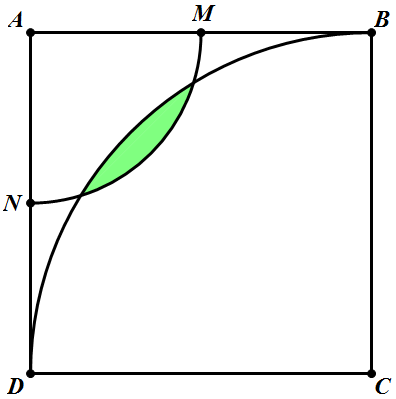# Area of a leaf

Geometry Level 5$ABCD$ is a square of side length 2, and $M$ and $N$ are the mid points of sides $AB$ and $AD$ respectively. Construct 2 circles with their center at $A$ and $C$ of radii 1 and 2 respectively.

Find the area of the green region.

×